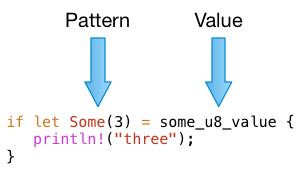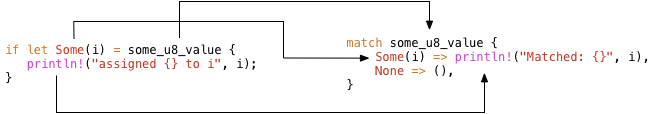# Learning Rust: If Let vs. MatchHuman languages have similar words with different
shades of meaning. Some computer languages do too.
(from: Wikimedia Commons)

This year I’ve decided to try to learn Rust. I’m fascinated by its ownership model for memory management; I’m curious what the claims about safety are all about; and, I love how it incorporates ideas from the functional programming world. But I haven’t gotten to all of that yet - I’m just getting started learning the basic syntax.

Learning a computer language is just like learning a human language. You have to try to read and write it everyday, even if just for a few minutes. You need to get to know some native speakers. And there’s no way around it: You need to learn the basic vocabulary of the language, word by word. To make things worse, our human languages usually have several words that mean the same thing. Which one should I use? Sometime only a native speaker will really know.

This week I was reading about if let and match in The Rust Programming Book (TRPL). I read that if let is really syntactic sugar for match:This intrigued me. The phrase “syntactic sugar” implies the two code snippets don’t only produce the same results, it means the compiler generates exactly the same code in each case.

Does the Rust compiler really generate exactly the same code for if let as it does for match? Read on to find out. Today I’ll start with a quick review of the syntax and meaning of if let and match. Then I’ll take a look at how Rust compiles if let and match, at what code it produces.

## If Let Compares a Pattern with a Value

The idea behind if let is that it compares a pattern with a value:In this example if let compares the pattern Some(3) with the value some_u8_value. If there’s a match, if let executes the println! code inside the block.

## If Let Also Assigns Values

if let assigns a value at the same time, when the pattern matches the value. This is the idea behind including the let keyword after if. This is more apparent if I rewrite the example using a variable i instead of 3. I'll also add a main function so I can execute the code:

```fn main() {
let some_u8_value = Some(3u8);
if let Some(i) = some_u8_value {
println!("assigned {} to i", i);
}
}```

When I saved this in a file called if-let.rs and ran it, I got:

```\$ rustc if-let.rs
\$ ./main
Assigned 3 to i```

if let “unwrapped” the option structure, and assigned the value 3 to the identifier i.

## Match: If Let’s Big Brother

As TRPL explains, I could also have written this using the match keyword, as follows:

```fn main() {
let some_u8_value = Some(3u8);
match some_u8_value {
Some(i) => println!("Matched: {}", i),
None => (),
}
}```

To write this all I had to do was move things around a bit in my if let code snippet from above:Because there was no else clause for the if let statement, I used None => () in match.

Saving this code in match.rs and running it I got the same result:

```\$ rustc match.rs
\$ ./main
Matched: 3```

## Mid-Level IR (MIR)

I was curious though: If these two code snippets are entirely equivalent, then the Rust compiler should generate exactly the same executable program when I compile them. In theory, therefore, I should be able to compare the two executable binaries to test whether TRPL’s statement about syntactic sugar is accurate. But comparing binary executables might not work. Likely there are timestamps or other ephemeral values encoded in the executable that would break the comparison. I decided to look for an easier way to test the compiler’s output.

Then I came across mid-level intermediate representation (MIR), described here on the Rust blog. MIR is an internal text language the rust compiler produces when you include the —emit-mir flag, like this:

`\$ rustc --emit mir if-let.rs`

With this option specified, rust generates a file called if-let.mir. Opening up this file, I see:

```// WARNING: This output format is intended for human consumers only
// and is subject to change without notice. Knock yourself out.
fn main() -> () {
let mut _0: ();                      // return pointer
scope 1 {
let _1: std::option::Option; // "some_u8_value" in scope 1 at src/if-let.rs:16:9: 16:22

etc…```

“Knock yourself out;” now I’m really intrigued!

## A First Look at MIR

I decided to compare the MIR text file the Rust compiler produced for the if let snippet vs. the match snippet. If Rust considers if let to be syntactic sugar for match, then the MIR representation of the two snippets should be the same.

But when I started reading the MIR code, I found the call to the println! macro generated a lot of verbose text:

```let mut _3: isize;
let mut _4: ();
let mut _5: std::fmt::Arguments;
let mut _6: &[&str];
let mut _7: &[&str; 2];
let mut _8: &[&str; 2];
let mut _9: &[std::fmt::ArgumentV1];
let mut _10: &[std::fmt::ArgumentV1; 1];
let mut _11: &[std::fmt::ArgumentV1; 1];
let mut _12: [std::fmt::ArgumentV1; 1];
let mut _13: (&u8,);
let mut _14: &u8;
let mut _16: std::fmt::ArgumentV1;
let mut _17: &u8;
let mut _18: fn(&u8, &mut std::fmt::Formatter<'_>) -> std::result::Result<(), std::fmt::Error>;```

All of this MIR pseudocode might confuse my comparison unnecessarily, so I decided to simplify my if let example by removing the println! call entirely. I rewrote the if let snippet like this (if-let.rs):

```fn main() {
let some_u8_value = Some(3u8);
if let Some(i) = some_u8_value {
let _ = i;
}
}```

And the match snippet like this (match.rs):

```fn main() {
let some_u8_value = Some(3u8);
match some_u8_value {
Some(i) => { let _ = i; }
None => ()
}
}```

I also noticed the MIR file contained many comments with line numbers:

```_2 = ((_1 as Some).0: u8);       // scope 3 at if-let.rs:3:17: 3:18
StorageLive(_5);                 // scope 3 at :2:27: 2:58
StorageLive(_6);                 // scope 3 at :3:18: 3:43```

I realized the line numbers would likely cause problems comparing one MIR file to another, so I removed all of the comments using sed:

```\$ rustc if-let.rs --emit mir
\$ cat if-let.mir | sed -e 's/\/\/.*\$//' > if-let.mir.nocomments```

This generates a new text file called if-let.mir.nocomments, which contains the same content as if-let.mir, but with no comments. And this command processes the match.rs file in the same way:

```\$ rustc match.rs --emit mir
\$ cat match.mir | sed -e 's/\/\/.*\$//' > match.mir.nocomments```

## Comparing MIR Files

Now I ran a simple diff command on the simplified MIR text files. If the compiler considers if let to be exactly the same as match then there should be no difference, then the output of diff should be empty.

But running diff I saw:

```\$ diff if-let.mir.nocomments match.mir.nocomments
19c19
<         switchInt(_3) -> [1isize: bb2, otherwise: bb1];
---
>         switchInt(_3) -> [0isize: bb1, otherwise: bb2];```

My two MIR files are almost identical; the MIR text Rust generates for if let is exactly the same as the MIR text Rust generates for match, except for line 19. I’ve almost proven the hypothesis that if let is syntactic sugar for match, but not quite.

Let’s take a close look at the MIR code around line 19 and try to understand what it means. Here’s a portion of if-let.mir.nocomments, produced by the Rust compiler from my if let code above:

```bb0: {
StorageLive(_1);
_1 = std::option::Option::Some(const 3u8,);
_3 = discriminant(_1);
switchInt(_3) -> [1isize: bb2, otherwise: bb1];
}

bb1: {
_0 = ();
goto -> bb3;
}

bb2: {
StorageLive(_2);
_2 = ((_1 as Some).0: u8);
_0 = ();
goto -> bb3;
}```

I don’t understand MIR syntax, but it’s not hard to guess what’s going on. Each of these “bb” blocks of code { … } probably represents a logical piece of my program.

The first block, bb0, seems to assign the value Some(3) to _1, and then calls discriminant(_1) and saves the “discriminant,” whatever that is, in _3. Finally, it tests whether the discriminant is 1. If the discriminant is 1 it jumps to bb2, or otherwise to bb1. So bb0 likely represents the if portion of my if let snippet, testing a condition:

`if let Some(i) = some_u8_value`

The bb1 block saves () in _0 and jumps to bb3. This likely represents the missing/default else clause of my if let statement.

And the bb2 block saves 3, the unwrapped value inside of Some(3), in _2 and jumps to bb3. Probably _2 is the variable i, and this block of MIR text represents the let portion of my if let snippet:

```let Some(i) = some_u8_value
let _ = i;```

Now let’s take a look at the match version, the contents of match.mir.nocomments. It’s entirely the same, except for the switchInt line:

```bb0: {
StorageLive(_1);
_1 = std::option::Option::Some(const 3u8,);
_3 = discriminant(_1);
switchInt(_3) -> [0isize: bb1, otherwise: bb2];
}```

Reading this carefully, I saw that actually it does mean the same thing: If the discriminant is 0, Rust calls the bb1 block, or otherwise the bb2 block.

So, summarizing, the if let snippet ran this pseudo-code:

If the discriminant is 1, call bb1, else bb2.

…and the match snippet ran this pseudo-code:

If the discriminant is 0, call bb2, else bb1.

So, in fact, the two versions use the same logic, assuming the value of discriminant is either 0 or 1. If discriminant = 0, Rust assumes the comparison was true and executes the match clause; if discriminant = 1, Rust executes the else clause.

Clearly the discriminant function is crucial - when I have time next, I’ll explore what discriminant means, where it’s implemented and how it works. Or if anyone from the Rust teams happens to read this, let us know.